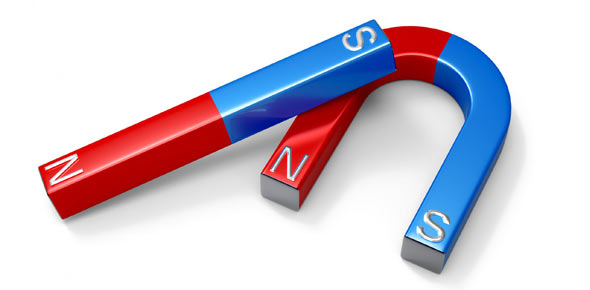# Simple Magnetism Terms Quiz

14 Questions | Total Attempts: 149SettingsCreate your own QuizTeam7c Magnetism quiz

• 1.
Any magnet has two ends, each one called a:
• A.

Magnetic field

• B.

Magnetic pole

• C.

Magnitized material

• D.

None of the above

• 2.
If two magnets were in this order: NS SN they would:
• A.

Repel

• B.

Attract

• C.

Do nothing

• 3.
The smallest particle of an element is known as an:
• A.

Proton

• B.

Neutron

• C.

Electron

• D.

Atom

• 4.
A particle that does not carry an electrical charge is a:
• A.

Proton

• B.

Neutron

• C.

Electron

• D.

Atom

• 5.
A ferromagnetic material is:
• A.

A material that shows strong magnetic effects

• B.

A material that shows weak magnetic effects

• C.

A material that shows no magnetic effects

• 6.
You can tell if an object is magnetic if the groupings of atoms:
• A.

Are scattered in all different directions

• B.

Are lined up in the same direction

• C.

Are lined up in opposite directions

• 7.
A magnet made of a material that keeps its magnetism is known as a:
• A.

• B.

Energizer bunny magnet

• C.

Permanent magnet

• D.

Temporary magnet

• 8.
How can you make a magnet temporarily lose its magnetism?
• A.

By heating it

• B.

By blowing on it

• C.

By hitting it very hard

• D.

Both a & c

• 9.
A tiny, negatively charged particle that usually exists in the outer region of an atom is called a:
• A.

Proton

• B.

Electron

• C.

Neutron

• D.

Nucleus

• 10.
The lines that map out the magnetic field around a magnet are:
• A.

Magnetic field lines

• B.

Magnetic pole lines

• C.

Magnetism

• 11.
One of about 100 basic materials that make up all matter.
• A.

Electron

• B.

Proton

• C.

Neutron

• D.

Element

• 12.
An object is not magnetized if:
• A.

The groupings of atoms are lined up

• B.

The groupings of atoms are scattered

• C.

The groupings of atoms are in the same direction

• 13.
If two magnets were in this order: NS NS they would:
• A.

Repel

• B.

Attract

• C.

Do nothing

• 14.
How many more weeks until break?
• A.

6

• B.

3

• C.

5

• D.

10

Related TopicsBack to top
×

Wait!
Here's an interesting quiz for you.